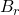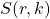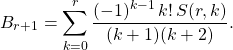# A new explicit formula for the Bernoulli numbers in terms of the Stirling numbers of the second kind

Sumit Kumar Jha
Notes on Number Theory and Discrete Mathematics
Print ISSN 1310–5132, Online ISSN 2367–8275
Volume 26, 2020, Number 2, Pages 148—151
DOI: 10.7546/nntdm.2020.26.2.148-151

## Details

### Authors and affiliations

Sumit Kumar JhaInternational Institute of Information Technology

### Abstract

Letdenote the Bernoulli numbers, anddenote the Stirling numbers of the second kind. We prove the following explicit formulaTo the best of our knowledge, the formula is new.

### Keywords

• Bernoulli numbers
• Stirling numbers of the second kind
• Riemann zeta function
• Polylogarithm function

• 11B68

### References

1. Fekih-Ahmed, L. (2012). On some explicit formulas for Bernoulli numbers and polynomials, preprint. Available online at: https://arxiv.org/abs/1106.5247.
2. Brychkov, Y. A., Marichev, O. I., & Savischenko, N. A. (2019). Handbook of Mellin Transforms, CRC Press, Boca Raton, FL.
3. Gould, H. W. (1972). Explicit formulas for Bernoulli numbers, Amer. Math. Monthly, 79 (1), 44–51.
4. Guo, B. N., Mező, I., & Qi, F. (2016). An explicit formula for the Bernoulli polynomials in terms of the r-Stirling numbers of the second kind, Rocky Mountain J. Math., 46 (6), 1919–1923.
5. Guo, B. N., & Qi, F. (2014). Some identities and an explicit formula for Bernoulli and Stirling numbers, J. Comput. Appl. Math., 255, 568–579.
6. Guo, B. N., & Qi, F. (2015). An explicit formula for Bernoulli numbers in terms of Stirling numbers of the second kind, J. Anal. Number Theory 3 (1), 27–30.
7. Jha, S. K. (2019). A congruence for the number of alternating permutations, preprint. Available at https://osf.io/yfz7h.
8. Jha, S. K. (2019). A new explicit formula for Bernoulli numbers involving the Euler number, Mosc. J. Comb. Number Theory, 8 (4), 385–387.
9. Jha, S. K. (2020). Two new explicit formulas for the Bernoulli numbers, Integers, 20, Article No. A21, 5 pp.
10. Jha, S. K. (2020). Two new explicit formulas for the even-indexed Bernoulli numbers, J. Integer Seq., 23 (2), Article No. 20.2.6.
11. Jha, S. K. (2020). An explicit formula for the Bernoulli polynomials in terms of the Stirling numbers of the second kind, preprint. Available online at: https://osf.io/n832v.
12. Landsburg, S. E. (2009). Stirling numbers and polylogarithms, preprint. Available online at: http://www.landsburg.com/query.pdf.
13. Qi, F., & Guo, B. N. (2014). Alternative proofs of a formula for Bernoulli numbers in terms of Stirling numbers, Analysis (Berlin), 34 (3), 311–317.
14. Quaintance, J., & Gould, H. W. (2015). Combinatorial Identities For Stirling Numbers: The Unpublished Notes of H. W. Gould, World Scientific..

## Cite this paper

Jha, S. K. (2020). A new explicit formula for the Bernoulli numbers in terms of the Stirling numbers of the second kind. Notes on Number Theory and Discrete Mathematics, 26 (2), 148-151, doi: 10.7546/nntdm.2020.26.2.148-151.